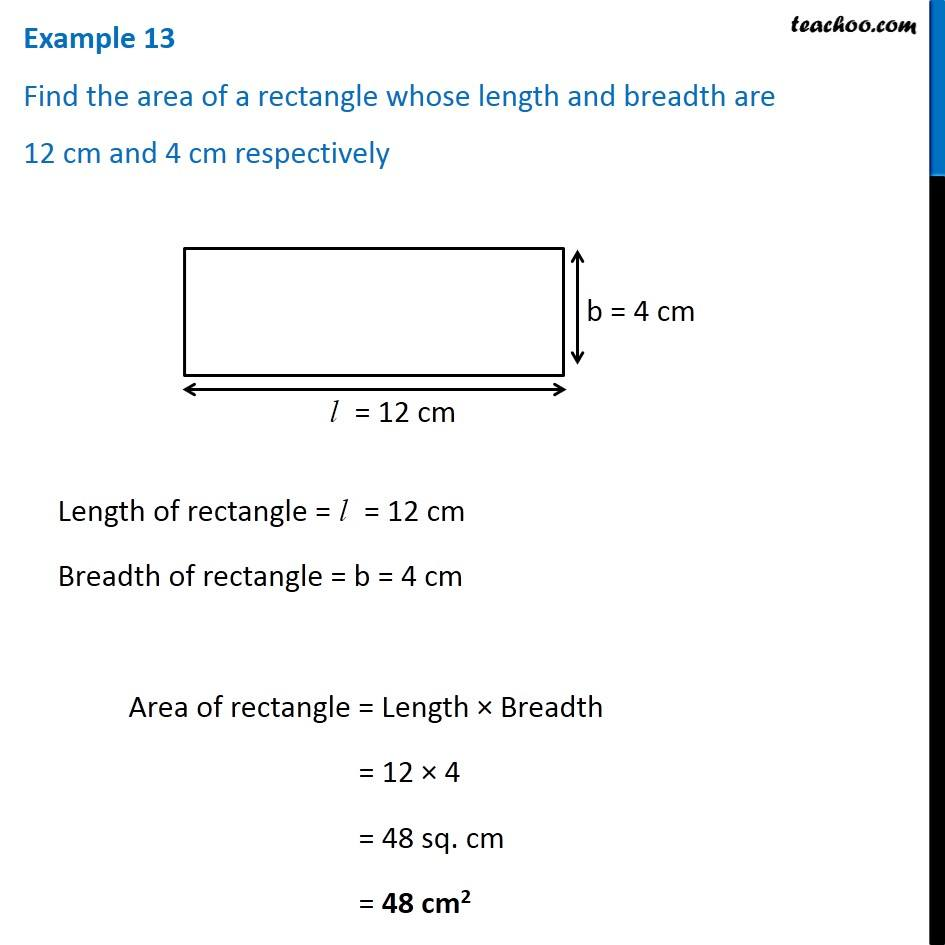Examples

Chapter 10 Class 6 Mensuration
Serial order wiseLearn in your speed, with individual attention - Teachoo Maths 1-on-1 Class

### Transcript

Example 13 Find the area of a rectangle whose length and breadth are 12 cm and 4 cm respectively Length of rectangle = l = 12 cm Breadth of rectangle = b = 4 cm Area of rectangle = Length × Breadth = 12 × 4 = 48 sq. cm = 48 cm2